# 5.3: Properties of Exponents and Scientific Notation

•• OpenStax
• OpenStax
$$\newcommand{\vecs}{\overset { \rightharpoonup} {\mathbf{#1}} }$$ $$\newcommand{\vecd}{\overset{-\!-\!\rightharpoonup}{\vphantom{a}\smash {#1}}}$$$$\newcommand{\id}{\mathrm{id}}$$ $$\newcommand{\Span}{\mathrm{span}}$$ $$\newcommand{\kernel}{\mathrm{null}\,}$$ $$\newcommand{\range}{\mathrm{range}\,}$$ $$\newcommand{\RealPart}{\mathrm{Re}}$$ $$\newcommand{\ImaginaryPart}{\mathrm{Im}}$$ $$\newcommand{\Argument}{\mathrm{Arg}}$$ $$\newcommand{\norm}{\| #1 \|}$$ $$\newcommand{\inner}{\langle #1, #2 \rangle}$$ $$\newcommand{\Span}{\mathrm{span}}$$ $$\newcommand{\id}{\mathrm{id}}$$ $$\newcommand{\Span}{\mathrm{span}}$$ $$\newcommand{\kernel}{\mathrm{null}\,}$$ $$\newcommand{\range}{\mathrm{range}\,}$$ $$\newcommand{\RealPart}{\mathrm{Re}}$$ $$\newcommand{\ImaginaryPart}{\mathrm{Im}}$$ $$\newcommand{\Argument}{\mathrm{Arg}}$$ $$\newcommand{\norm}{\| #1 \|}$$ $$\newcommand{\inner}{\langle #1, #2 \rangle}$$ $$\newcommand{\Span}{\mathrm{span}}$$$$\newcommand{\AA}{\unicode[.8,0]{x212B}}$$

##### Learning Objectives

By the end of this section, you will be able to:

• Simplify expressions using the properties for exponents
• Use the definition of a negative exponent
• Use scientific notation
##### Note

Before you get started, take this readiness quiz.

1. Simplify: $$(−2)(−2)(−2)$$.
If you missed this problem, review [link].
2. Simplify: $$\dfrac{8x}{24y}$$.
If you missed this problem, review [link].
3. Name the decimal $$(−2.6)(4.21)$$.
If you missed this problem, review [link].

## Simplify Expressions Using the Properties for Exponents

Remember that an exponent indicates repeated multiplication of the same quantity. For example, in the expression $$a^m$$, the exponent $$m$$ tells us how many times we use the base $$a$$ as a factor.

$a^{m}= \underbrace{a \cdot a \cdot a \cdot \ldots \cdot a}_{\color{cyan}{\text{m factors}}} \nonumber$

For example

$(-9)^{5}= \underbrace{ (-9)\cdot (-9)\cdot (-9)\cdot (-9) \cdot (-9)}_{\color{cyan}{\text{5 factors}}} \nonumber$

Let’s review the vocabulary for expressions with exponents.

##### DEFINITION: EXPONENTIAL NOTATIONThis is read $$a$$ to the $$m^{th}$$ power.

In the expression $$a^m$$, the exponent $$m$$ tells us how many times we use the base $$a$$ as a factor.

When we combine like terms by adding and subtracting, we need to have the same base with the same exponent. But when you multiply and divide, the exponents may be different, and sometimes the bases may be different, too.

First, we will look at an example that leads to the Product Property.

 $$x^{2} \cdot x^{3}$$ What does this mean?$$\underbrace{x \cdot x}_{2 factors} \color{cyan}{\cdot} \underbrace{\color{black}{} x\cdot x \cdot x}_{3 factors}$$ $$x^{5}$$

Notice that 5 is the sum of the exponents, 2 and 3. We see $$x_2 \cdot x_3$$ is $$x^{2+3}$$ or $$x^5$$.

The base stayed the same and we added the exponents. This leads to the Product Property for Exponents.

##### DEFINITION: PRODUCT PROPERTY FOR EXPONENTS

If a is a real number and $$m$$ and $$n$$ are integers, then

$a^m·a^n=a^{m+n} \nonumber$

To multiply with like bases, add the exponents.

##### Example $$\PageIndex{1}$$

Simplify each expression:

1. $$y^5·y^6$$
2. $$2^x·2^{3x}$$
3. $$2a^7·3a$$.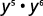Use the Product Property, $$a^m·a^n=a^{m+n}$$.Simplify.Use the Product Property, $$a^m·a^n=a^{m+n}$$.Simplify.Rewrite, $$a=a^1$$.Use the Commutative Property and use the Product Property, $$a^m·a^n=a^{m+n}$$.Simplify.Add the exponents, since bases are the same.Simplify.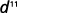##### Example $$\PageIndex{2}$$

Simplify each expression:

1. $$b^9·b^8$$
2. $$4^{2x}·4^x$$
3. $$3p^5·4p$$
4. $$x^6·x^4·x^8$$.

ⓐ $$b^{17}$$ ⓑ $$4^{3x}$$ ⓒ $$12p^6$$
ⓓ $$x^{18}$$

##### Example $$\PageIndex{3}$$

Simplify each expression:

1. $$x^{12}·x4$$
2. $$10·10^x$$
3. $$2z·6z^7$$
4. $$b^5·b^9·b^5$$.

$$x^{16}$$

$$10^{x+1}$$

$$12z^8$$

$$b^{19}$$

Now we will look at an exponent property for division. As before, we’ll try to discover a property by looking at some examples.

 Consider $$\dfrac{x^5}{x^2}$$ and $$\dfrac{x^2}{x^3}$$ What do they mean? $$\dfrac{x·x·x·x·x}{x·x}$$ $$\dfrac{x·x}{x·x·x}$$ Use the Equivalent Fractions Property. $$\dfrac{\cancel{x}·\cancel{x}·x·x·x}{\cancel{x}·\cancel{x}}$$ $$\dfrac{\cancel{x}·\cancel{x}·1}{\cancel{x}·\cancel{x}·x}$$ Simplify. $$x^3$$ $$\dfrac{1}{x}$$

Notice, in each case the bases were the same and we subtracted exponents. We see $$\dfrac{x^5}{x^2}$$ is $$x^{5−2}$$ or $$x^3$$. We see $$\dfrac{x^2}{x^3}$$ is or $$\dfrac{1}{x}$$. When the larger exponent was in the numerator, we were left with factors in the numerator. When the larger exponent was in the denominator, we were left with factors in the denominator--notice the numerator of 1. When all the factors in the numerator have been removed, remember this is really dividing the factors to one, and so we need a 1 in the numerator. $$\dfrac{\cancel{x}}{\cancel{x}}=1$$. This leads to the Quotient Property for Exponents.

##### Definition: QUOTIENT PROPERTY FOR EXPONENTS

If $$a$$ is a real number, $$a \neq 0$$, and $$m$$ and $$n$$ are integers, then

$\begin{array} {lllll} {\dfrac{a^m}{a^n}=a^{m−n},} &{m>n} &{\text{and}} &{\dfrac{a^m}{a^n}=\dfrac{1}{a^{n−m}},} &{n>m} \\ \nonumber \end{array}$

##### Example $$\PageIndex{4}$$

Simplify each expression:

1. $$\dfrac{x^9}{x^7}$$
2. $$\dfrac{3^{10}}{3^2}$$
3. $$\dfrac{b^8}{b^{12}}$$
4. $$\dfrac{7^3}{7^5}$$.

To simplify an expression with a quotient, we need to first compare the exponents in the numerator and denominator.

 Since $$9>7$$, there are more factors of $$x$$ in the numerator.Use Quotient Property, $$\dfrac{a^m}{a^n}=a^{m−n}$$.Simplify.Since $$10>2$$, there are more factors of $$3$$ in the numerator.Use Quotient Property, $$\dfrac{a^m}{a^n}=a^{m−n}$$.Simplify.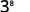Notice that when the larger exponent is in the numerator, we are left with factors in the numerator.

 Since $$12>8$$, there are more factors of bb in the denominator.Use Quotient Property, $$\dfrac{a^m}{a^n}=a^{m−n}$$.Simplify.Since $$5>3$$, there are more factors of $$3$$ in the denominator.Use Quotient Property, $$\dfrac{a^m}{a^n}=a^{m−n}$$.Simplify.Simplify.Notice that when the larger exponent is in the denominator, we are left with factors in the denominator.

##### Example $$\PageIndex{5}$$

Simplify each expression:

1. $$\dfrac{x^{15}}{x^{10}}$$
2. $$\dfrac{6^{14}}{6^5}$$
3. $$\dfrac{x^{18}}{x^{22}}$$
4. $$\dfrac{12^{15}}{12^{30}}$$.

ⓐ $$x^5$$

ⓑ $$6^9$$

ⓒ $$\dfrac{1}{x^4}$$
ⓓ $$\dfrac{1}{12^{15}}$$

##### Example $$\PageIndex{6}$$

Simplify each expression:

1. $$\dfrac{y^{43}}{y^{37}}$$
2. $$\dfrac{10^{15}}{10^{7}}$$
3. $$\dfrac{m^7}{m^{15}}$$
4. $$\dfrac{9^8}{9^{19}}$$.

ⓐ $$y_6$$

ⓑ $$108$$

ⓒ $$1m8$$
ⓓ $$\dfrac{1}{9^{11}}$$

A special case of the Quotient Property is when the exponents of the numerator and denominator are equal, such as an expression like $$\dfrac{a^m}{a^m}$$. We know, $$\dfrac{x}{x}=1$$, for any $$x(x\neq 0)$$ since any number divided by itself is 1.

The Quotient Property for Exponents shows us how to simplify $$\dfrac{a^m}{a^m}$$. when $$m>n$$ and when n<mn<m by subtracting exponents. What if $$m=n$$? We will simplify $$\dfrac{a^m}{a^m}$$ in two ways to lead us to the definition of the Zero Exponent Property. In general, for $$a \neq 0$$:We see $$\dfrac{a^m}{a^m}$$ simplifies to $$a^0$$ and to 1. So $$a^0=1$$. Any non-zero base raised to the power of zero equals $$1$$.

##### DEFINITION: ZERO EXPONENT PROPERTY

If $$a$$ is a non-zero number, then $$a^0=1$$.

If $$a$$ is a non-zero number, then $$a$$ to the power of zero equals $$1$$.

Any non-zero number raised to the zero power is $$1$$.

In this text, we assume any variable that we raise to the zero power is not zero.

##### ExAMPLE $$\PageIndex{7}$$

Simplify each expression: ⓐ $$9^0$$ ⓑ $$n^0$$.

The definition says any non-zero number raised to the zero power is $$1$$.

ⓐ Use the definition of the zero exponent. $$9^0 = 1$$

ⓑ Use the definition of the zero exponent. $$n^0 = 1$$

To simplify the expression $$n$$ raised to the zero power we just use the definition of the zero exponent. The result is $$1$$.

##### Example $$\PageIndex{8}$$

Simplify each expression: ⓐ $$11^0$$ ⓑ $$q^0$$.

ⓐ 1

ⓑ 1

##### Example $$\PageIndex{9}$$

Simplify each expression: ⓐ $$23^0$$ ⓑ $$r^0$$.

ⓐ 1

ⓑ 1

## Use the Definition of a Negative Exponent

We saw that the Quotient Property for Exponents has two forms depending on whether the exponent is larger in the numerator or the denominator. What if we just subtract exponents regardless of which is larger?

Let’s consider $$\dfrac{x^2}{x^5}$$. We subtract the exponent in the denominator from the exponent in the numerator. We see $$\dfrac{x^2}{x^5}$$ is $$x^{2−5}$$ or $$x^{−3}$$.

We can also simplify $$\dfrac{x^2}{x^5}$$ by dividing out common factors:This implies that $$x^{−3}=\dfrac{1}{x^3}$$ and it leads us to the definition of a negative exponent. If n is an integer and $$a\neq 0$$, then $$a^{−n}=\dfrac{1}{a^n}$$.

Let’s now look at what happens to a fraction whose numerator is one and whose denominator is an integer raised to a negative exponent.

$$\begin{array} {ll} {} &{\dfrac{1}{a^{-n}}} \\ {} &{} \\ {\text{Use the definition of a negative exponent, } a^{-n}= \dfrac{1}{a^n}} &{\dfrac{1}{\dfrac{1}{a^n}}} \\ {} &{} \\ {\text{Simplify the complex fraction.}} &{1·\dfrac{a^n}{1}} \\ {} &{} \\ {\text{Multiply.}} &{a^n} \\ \end{array}$$

This implies $$\dfrac{1}{a^{−n}}=a^n$$ and is another form of the definition of Properties of Negative Exponents.

##### DEFINITION: PROPERTIES OF NEGATIVE EXPONENTS

If $$n$$ is an integer and $$a\neq 0$$, then $$a^{−n}=\dfrac{1}{a^n}$$ or $$\dfrac{1}{a^{−n}}=a^n$$.

The negative exponent tells us we can rewrite the expression by taking the reciprocal of the base and then changing the sign of the exponent.

Any expression that has negative exponents is not considered to be in simplest form. We will use the definition of a negative exponent and other properties of exponents to write the expression with only positive exponents.

For example, if after simplifying an expression we end up with the expression $$x^{−3}$$, we will take one more step and write $$\dfrac{1}{x^3}$$. The answer is considered to be in simplest form when it has only positive exponents.

##### Example $$\PageIndex{10}$$

Simplify each expression: ⓐ $$x^{−5}$$ ⓑ $$10^{−3}$$ ⓒ $$\dfrac{1}{y^{−4}}$$ ⓓ $$13^{−2}$$.

$$\begin{array} {ll} {} &{x^{−5}} \\ {\text{Use the definition of a negative exponent, } a^{−n}=\dfrac{1}{a^n}.} &{\dfrac{1}{x^5}} \\ \end{array}$$

$$\begin{array} {ll} {} &{10^{-3}} \\ {\text{Use the definition of a negative exponent, }a^{−n}=\dfrac{1}{a^n}.} &{\dfrac{1}{10^3}} \\ {\text{Simplify.}} &{\dfrac{1}{1000}} \\ \end{array}$$

$$\begin{array} {ll} {} &{\dfrac{1}{y^{-4}}} \\ {\text{Use the property of a negative exponent, } \dfrac{1}{a^{−n}}=a^n.} &{y^4} \\ \end{array}$$

$$\begin{array} {ll} {} &{\dfrac{1}{3^{-2}}} \\ {\text{Use the property of a negative exponent, } \dfrac{1}{a^{−n}}=a^n.} &{3^2} \\ {\text{Simplify.}} &{9} \\ \end{array}$$

##### Example $$\PageIndex{11}$$

Simplify each expression: ⓐ $$z^{−3}$$ ⓑ $$10^{−7}$$ ⓒ $$\dfrac{1}{p^{−8}}$$ ⓓ $$\dfrac{1}{4^{−3}}$$.

ⓐ $$\dfrac{1}{z^3}$$ ⓑ $$\dfrac{1}{10^7}$$ ⓒ $$p^8$$ ⓓ $$64$$

##### Example $$\PageIndex{12}$$

Simplify each expression: ⓐ $$n^{−2}$$ ⓑ $$10^{−4}$$ ⓒ $$\dfrac{1}{q^{−7}}$$ ⓓ $$\dfrac{1}{2^{−4}}$$.

ⓐ $$\dfrac{1}{n^2}$$ ⓑ $$\dfrac{1}{10,000}$$ ⓒ $$q^7$$
ⓓ $$16$$

Suppose now we have a fraction raised to a negative exponent. Let’s use our definition of negative exponents to lead us to a new property.

$$\begin{array} {ll} {} &{\left( \dfrac{3}{4} \right)^{-2}} \\ {} &{} \\ {\text{Use the definition of a negative exponent, } a^{−n}=\dfrac{1}{a^n}.} &{\dfrac{1}{\left( \dfrac{3}{4} \right)^{2}}} \\ {} &{} \\ {\text{Simplify the denominator.}} &{\dfrac{1}{\dfrac{9}{16}}} \\{} &{} \\ {\text{Simplify the complex fraction.}} &{\dfrac{16}{9}} \\ {} &{} \\ {\text{But we know that }\dfrac{16}{9}\text{ is } \left( \dfrac{4}{3} \right)^{2}.} &{} \\ {\text{This tells us that}} &{\left( \dfrac{3}{4} \right)^{-2} = \left( \dfrac{4}{3} \right)^{2}} \\ \end{array}$$

To get from the original fraction raised to a negative exponent to the final result, we took the reciprocal of the base—the fraction—and changed the sign of the exponent.

This leads us to the Quotient to a Negative Power Property.

##### QUOTIENT TO A NEGATIVE POWER PROPERTY

If $$a$$ and $$b$$ are real numbers, $$a\neq 0$$, $$b\neq 0$$ and $$n$$ is an integer, then

$\left(\dfrac{a}{b}\right)^{−n}=\left(\dfrac{b}{a}\right)^n \nonumber$.

##### Example $$\PageIndex{13}$$

Simplify each expression: ⓐ $$\left( \dfrac{5}{7} \right)^{−2}$$ ⓑ $$\left( −\dfrac{x}{y} \right)^{−3}$$.

$$\begin{array} {ll} {} &{\left( \dfrac{5}{7}\right)^{-2}} \\ {\text{Use the Quotient to a Negative Exponent Property, } \left(\dfrac{a}{b} \right)^{−n}= \left( \dfrac{b}{a} \right)^n.} &{} \\ {\text{Take the reciprocal of the fraction and change the sign of the exponent.}} &{\left( \dfrac{7}{5}\right)^2} \\ {\text{Simplify.}} &{\dfrac{49}{25}} \\ \end{array}$$

$$\begin{array} {ll} {} &{\left( -\dfrac{x}{y}\right)^{-3}} \\ {\text{Use the Quotient to a Negative Exponent Property, } \left(\dfrac{a}{b} \right)^{−n}= \left( \dfrac{b}{a} \right)^n.} &{} \\ {\text{Take the reciprocal of the fraction and change the sign of the exponent.}} &{\left( -\dfrac{y}{x}\right)^3} \\ {\text{Simplify.}} &{-\dfrac{y^3}{x^3}} \\ \end{array}$$

##### Example $$\PageIndex{14}$$

Simplify each expression: ⓐ $$\left(\dfrac{2}{3}\right)^{−4}$$ ⓑ $$\left(−\dfrac{m}{n}\right)^{−2}$$.

ⓐ $$\dfrac{81}{16}$$ ⓑ $$\dfrac{n^2}{m^2}$$

##### Example $$\PageIndex{15}$$

Simplify each expression: ⓐ $$\left(\dfrac{3}{5}\right)^{−3}$$ ⓑ $$\left(−\dfrac{a}{b}\right)^{−4}$$.

ⓐ $$\dfrac{125}{27}$$ ⓑ $$\dfrac{b^4}{a^4}$$

Now that we have negative exponents, we will use the Product Property with expressions that have negative exponents.

##### Example $$\PageIndex{16}$$

Simplify each expression: ⓐ $$z^{−5}·z^{−3}$$ ⓑ $$(m^4n^{−3})(m^{−5}n^{−2})$$ ⓒ $$(2x^{−6}y^8)(−5x^5y^{−3})$$.

$$\begin{array} {ll} {} &{z^{−5}·z^{−3}} \\ {\text{Add the exponents, since the bases are the same.}} &{z^{−5−3}} \\ {\text{Simplify.}} &{z^{−8}} \\ {\text{Use the definition of a negative exponent.}} &{\dfrac{1}{z^8}} \\ \end{array}$$

$$\begin{array} {ll} {} &{(m^4n^{−3})(m^{−5}n^{−2})} \\ {\text{Use the Commutative Property to get like}} &{} \\ {\text{bases together.}} &{m^4m^{−5}·n^{−2}n^{−3}} \\ {\text{Add the exponents for each base.}} &{m^{−1}·n^{−5}} \\ {\text{Take reciprocals and change the signs of the exponents.}} &{\dfrac{1}{m^1}·\dfrac{1}{n^5}} \\ {\text{Simplify.}} &{\dfrac{1}{mn^5}} \\ \end{array}$$

$$\begin{array} {ll} {} &{(2x^{−6}y^8)(−5x^5y^{−3})} \\ {\text{Rewrite with the like bases together.}} &{2(−5)·(x^{−6}x^5)·(y^8y^{−3})} \\ {\text{Multiply the coefficients and add the exponents}} &{} \\ {\text{of each variable.}} &{−10·x^{−1}·y5} \\ {\text{Use the definition of a negative exponent,} a^{−n}=\dfrac{1}{a^n}.} &{−10·\dfrac{1}{x}·y^5} \\ {\text{Simplify.}} &{−10y^5x} \\ \end{array}$$

##### Example $$\PageIndex{17}$$

Simplify each expression:

ⓐ $$z^{−4}·z^{−5}$$ ⓑ $$(p^6q^{−2})(p^{−9}q^{−1})$$ ⓒ $$(3u^{−5}v^7)(−4u^4v^{−2})$$.

ⓐ $$\dfrac{1}{z^9}$$ ⓑ $$\dfrac{1}{p^3q^3}$$ ⓒ $$−\dfrac{12v^5}{u}$$

##### Example $$\PageIndex{18}$$

Simplify each expression:

ⓐ $$c^{−8}·c^{−7}$$ ⓑ $$(r^5s^{−3})(r^{−7}s^{−5})$$ ⓒ $$(−6c^{−6}d^4)(−5c^{−2}d^{−1})$$.

ⓐ $$\dfrac{1}{c^15}$$ ⓑ $$\dfrac{1}{r^2s^8}$$ ⓒ $$\dfrac{30d^3}{c^8}$$

Now let’s look at an exponential expression that contains a power raised to a power. See if you can discover a general property.

$$\begin{array} {ll} {} &{(x^2)^3} \\ {\text{What does this mean?}} &{x^2·x^2·x^2} \\ \end{array}$$

 How many factors altogether?So we haveNotice the 6 is the product of the exponents, 2 and 3. We see that $$(x^2)^3$$ is $$x^{2·3}$$ or $$x^6$$.

We multiplied the exponents. This leads to the Power Property for Exponents.

##### DEFINITION: POWER PROPERTY FOR EXPONENTS

If $$a$$ is a real number and $$m$$ and $$n$$ are integers, then

$(a^m)^n=a^{m·n} \nonumber$

To raise a power to a power, multiply the exponents.

##### Example $$\PageIndex{19}$$

Simplify each expression: ⓐ $$(y^5)^9$$ ⓑ $$(4^4)^7$$ ⓒ $$(y^3)^6(y^5)^4$$.Use the Power Property, $$(a^m)^n=a^{m·n}$$.Simplify.Use the Power Property.Simplify.$$\begin{array} {ll} {} &{(y^3)^6(y^5)^4} \\ {\text{Use the Power Property.}} &{y^{18}·y^{20}} \\ {\text{Add the exponents.}} &{y^{38}} \\ \end{array}$$

##### Example $$\PageIndex{20}$$

Simplify each expression: ⓐ $$(b^7)^5$$ ⓑ $$(5^4)^3$$ ⓒ $$(a^4)^5(a^7)^4$$.

ⓐ $$b^{35}$$ ⓑ $$5^{12}$$ ⓒ $$a^{48}$$

##### Example $$\PageIndex{21}$$

Simplify each expression: ⓐ $$(z^6)^9$$ ⓑ $$(3^7)^7$$ ⓒ $$(q^4)^5(q^3)^3$$.

ⓐ $$z^{54}$$ ⓑ $$3^{49}$$ ⓒ $$q^{29}$$

We will now look at an expression containing a product that is raised to a power. Can you find this pattern?

$$\begin{array} {ll} {} &{(2x)^3} \\ {\text{What does this mean?}} &{2x·2x·2x} \\ {\text{We group the like factors together.}} &{2·2·2·x·x·x} \\ {\text{How many factors of 2 and of }}x &{2^3·x^3} \\ \end{array}$$

Notice that each factor was raised to the power and $$(2x)^3$$ is $$2^3·x^3$$.

The exponent applies to each of the factors! This leads to the Product to a Power Property for Exponents.

##### DEFINITION: PRODUCT TO A POWER PROPERTY FOR EXPONENTS

If $$a$$ and $$b$$ are real numbers and $$m$$ is a whole number, then

$(ab)^m=a^mb^m \nonumber$

To raise a product to a power, raise each factor to that power.

##### Example $$\PageIndex{22}$$

Simplify each expression: ⓐ $$(−3mn)^3$$ ⓑ $$(−4a^2b)^0$$ ⓒ $$(6k^3)^{−2}$$ ⓓ $$(5x^{−3})^2$$.Use Power of a Product Property, $$(ab)^m=a^mb^m$$.Simplify.$$\begin{array} {ll} {} &{(−4a^2b)^0} \\ {\text{Use Power of a Product Property, }(ab)^m=a^mb^m.} &{(−4)^0(a^2)^0(b)^0} \\ {\text{Simplify.}} &{1·1·1} \\ {\text{Multiply.}} &{1} \\ \end{array}$$

$$\begin{array} {ll} {} &{(6k^3)^{−2}} \\ {\text{Use Power of a Product Property, }(ab)^m=a^mb^m.} &{(6)^{−2}(k^3)^{−2}} \\ {\text{Use the Power Property, }(a^m)^n=a^{m·n}.} &{6^{−2}k^{−6}} \\ {\text{Use the Definition of a negative exponent, }a^{−n}=\dfrac{1}{a^n}.} &{\dfrac{1}{6^2}·\dfrac{1}{k^6}} \\ {\text{Simplify.}} &{\dfrac{1}{36k^6}} \\ \end{array}$$

$$\begin{array} {ll} {} &{(5x^{−3})^2} \\ {\text{Use Power of a Product Property, }(ab)^m=a^mb^m.} &{5^2(x^{−3})^2} \\ {\text{Simplify.}} &{25·x^{−6}} \\ {\text{Rewrite }x−6 \text{using, }a^{−n}=\text{1}{a^n}.} &{25·\dfrac{1}{x^6}} \\ {\text{Simplify.}} &{\dfrac{25}{x^6}} \\ \end{array}$$

##### Example $$\PageIndex{23}$$

Simplify each expression: ⓐ $$(2wx)^5$$ ⓑ $$(−11pq3)^0$$ ⓒ $$(2b^3)^{−4}$$ ⓓ $$(8a^{−4})^2$$.

ⓐ $$32w^5x^5$$ ⓑ 1 ⓒ $$\dfrac{1}{16b^{12}}$$
ⓓ $$\dfrac{64}{a^8}$$

##### Example $$\PageIndex{24}$$

Simplify each expression: ⓐ $$(−3y)^3$$ ⓑ $$(−8m^2n^3)^0$$ ⓒ $$(−4x^4)^{−2}$$ ⓓ $$(2c^{−4})^3$$.

ⓐ $$−27y^3$$ ⓑ 1 ⓒ $$\dfrac{1}{16x^8}$$
ⓓ $$8c^{12}$$

Now we will look at an example that will lead us to the Quotient to a Power Property.

$$\begin{array} {ll} {} &{\left( \dfrac{x}{y}\right)^3} \\ {\text{This means}} &{\dfrac{x}{y}·\dfrac{x}{y}·\dfrac{x}{y}} \\ {\text{Multiply the fractions.}} &{\dfrac{x·x·x}{y·y·y}} \\ {\text{Write with exponents.}} &{\dfrac{x^3}{y^3}} \\ \end{array}$$

Notice that the exponent applies to both the numerator and the denominator.

We see that $$\left(\dfrac{x}{y}\right)^3$$ is $$\dfrac{x^3}{y^3}$$.

This leads to the Quotient to a Power Property for Exponents.

##### DEFINITION: QUOTIENT TO A POWER PROPERTY FOR EXPONENTS

If $$a$$ and $$b$$ are real numbers, $$b\neq 0$$, and $$m$$ is an integer, then

$\left(\dfrac{a}{b}\right)^m=\dfrac{a^m}{b^m} \nonumber$

To raise a fraction to a power, raise the numerator and denominator to that power.

##### Example $$\PageIndex{25}$$

Simplify each expression:

ⓐ $$\left(\dfrac{b}{3}\right)^4$$ ⓑ $$\left(\dfrac{k}{j}\right)^{−3}$$ ⓒ $$\left(\dfrac{2xy^2}{z}\right)^3$$ ⓓ $$\left(\dfrac{4p^{−3}}{q^2}\right)^2$$.Use Quotient to a Power Property, $$(ab)^m=a^mb^m$$.Simplify.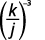Raise the numerator and denominator to the power.Use the definition of negative exponent.Multiply.$$\begin{array} {ll} {} &{\left(\dfrac{2xy^2}{z}\right)^3} \\ {\text{Use Quotient to a Power Property, }\left(\dfrac{a}{b}\right)^m=\dfrac{a^m}{b^m}.} &{\dfrac{(2xy^2)^3}{z^3}} \\ {\text{Use the Product to a Power Property, }(ab)^m=a^mb^m.} &{\dfrac{8x^3y^6}{z^3}} \\ \end{array}$$

$$\begin{array} {ll} {} &{\left(\dfrac{4p^{−3}}{q^2}\right)^2} \\ {\text{Use Quotient to a Power Property, }\left(\dfrac{a}{b}\right)^m=\dfrac{a^m}{b^m}.} &{\dfrac{(4p^{−3})^2}{(q^2)^2}} \\ {\text{Use the Product to a Power Property, }(ab)^m=a^mb^m.} &{\dfrac{4^2(p^{−3})^2}{(q^2)^2}} \\ {\text{Simplify using the Power Property, }(a^m)^n=a^{m·n}.} &{\dfrac{16p^{−6}}{q^4}} \\ {\text{Use the definition of negative exponent.}} &{\dfrac{16}{q^4}·\dfrac{1}{p^6}} \\ {\text{Simplify.}} &{\dfrac{16}{p^6q^4}} \\ \end{array}$$

##### Example $$\PageIndex{26}$$

Simplify each expression:

ⓐ $$\left(\dfrac{p}{10}\right)^4$$ ⓑ $$\left(\dfrac{m}{n}\right)^{−7}$$ ⓒ$$\left(\dfrac{3ab^3}{c^2}\right)^4$$ ⓓ $$\left(\dfrac{3x^{−2}}{y^3}\right)^3$$.

ⓐ $$\dfrac{p^4}{10000}$$ ⓑ $$\dfrac{n^7}{m^7}$$
ⓒ $$\dfrac{81a^4b^{12}}{c^8}$$ ⓓ $$\dfrac{27}{x^6y^9}$$

##### Example $$\PageIndex{27}$$

Simplify each expression:

ⓐ $$\left(\dfrac{−2}{q}\right)^3$$ ⓑ $$\left(\dfrac{w}{x}\right)^{−4}$$ ⓒ $$\left(\dfrac{xy^3}{3z^2}\right)^2$$ ⓓ $$\left(\dfrac{2m^{−2}}{n^{−2}}\right)^3$$.

ⓐ $$\dfrac{−8}{q^3}$$ ⓑ $$\dfrac{x^4}{w^4}$$ ⓒ $$\dfrac{x^2y^6}{9z^4}$$
ⓓ $$\dfrac{8n^6}{m^6}$$

We now have several properties for exponents. Let’s summarize them and then we’ll do some more examples that use more than one of the properties.

##### DEFINITION: SUMMARY OF EXPONENT PROPERTIES

If $$a$$ and $$b$$ are real numbers, and $$m$$ and $$n$$ are integers, then

Property Description
Product Property $$a^m·a^n=a^{m+n}$$
Power Property $$(a^m)^n=a^{m·n}$$
Product to a Power $$(ab)^n=a^nb^n$$
Quotient Property $$\dfrac{a^m}{a^n}=a^{m−n},a\neq 0$$
Zero Exponent Property $$a^0=1,a \neq 0$$
Quotient to a Power Property $$\left(\dfrac{a}{b}\right)^m=\dfrac{a^m}{b^m},b \neq 0$$
Properties of Negative Exponents $$a^{−n}=\dfrac{1}{a^n}$$ and $$\dfrac{1}{a^{−n}}=a^n$$
Quotient to a Negative Exponent $$\left(\dfrac{a}{b}\right)^{−n}=\left(\dfrac{b}{a}\right)^n$$
##### Example $$\PageIndex{28}$$

Simplify each expression by applying several properties:

ⓐ $$(3x^2y)^4(2xy^2)^3$$ ⓑ $$\dfrac{(x^3)^4(x^{−2})^5}{(x^6)^5}$$ ⓒ $$\left(\dfrac{2xy^2}{x^3y^{−2}}\right)^2 \left(\dfrac{12xy^3}{x^3y^{−1}}\right)^{−1}$$.

$$\begin{array} {ll} {} &{(3x^2y)^4(2xy^2)^3} \\ {} &{} \\ {\text{Use the Product to a Power Property, }(ab)^m=a^mb^m.} &{(3^4x^8y^4)(2^3x^3y^6)} \\ {} &{} \\ {\text{Simplify.}} &{(81x^8y^4)(8x^3y^6)} \\ {} &{} \\ {\text{Use the Commutative Property.}} &{81·8·x^8·x^3·y^4·y^6} \\ {} &{} \\ {\text{Multiply the constants and add the exponents.}} &{648x^{11}y^{10}} \\ \end{array}$$

$$\begin{array} {ll} {} &{\dfrac{(x^3)^4(x^{−2})^5}{(x^6)^5}} \\ {\text{Use the Power Property, }(a^m)^n=a^{m·n}.} &{(x^{12})(x^{−10})(x^{30})} \\ {\text{Add the exponents in the numerator.}} &{\dfrac{x^2}{x^{30}}} \\ {\text{Use the Quotient Property, }\dfrac{a^m}{a^n}=\dfrac{1}{a^{n−m}}.} &{\dfrac{1}{x^{28}}} \\ \end{array}$$

$$\begin{array} {ll} {} &{\left(\dfrac{2xy^2}{x^3y^{−2}}\right)^2 \left(\dfrac{12xy^3}{x^3y^{−1}}\right)^{−1}} \\ {\text{Simplify inside the parentheses first.}} &{\left(\dfrac{2y^4}{x^2}\right)^2\left(\dfrac{12y^4}{x^2}\right)^{−1}} \\ {\text{Use the Quotient to a Power Property, }\left(\dfrac{a}{b}\right)^m=\dfrac{a^m}{b^m}.} &{\dfrac{(2y^4)^2}{(x^2)^2}\dfrac{(12y^4)^{−1}}{(x^2)^{−1}}} \\ {\text{Use the Product to a Power Property, }(ab)^m=a^mb^m.} &{\dfrac{4y^8}{x^4}·\dfrac{12^{−1}y^{−4}}{x^{−2}}} \\ {\text{Simplify.}} &{\dfrac{4y^4}{12x^2}} \\ {\text{Simplify.}} &{\dfrac{y^4}{3x^2}} \\ \end{array}$$

##### Example $$\PageIndex{29}$$

Simplify each expression:

ⓐ $$(c^4d^2)^5(3cd^5)^4$$ ⓑ $$\dfrac{(a^{−2})^3(a^2)^4}{(a^4)^5}$$ ⓒ $$\left(\dfrac{3xy^2}{x^2y^{−3}}\right)^2$$

ⓐ $$81c^{24}d^{30}$$ ⓑ $$\dfrac{1}{a^{18}}$$
ⓒ $$\dfrac{9y^{10}}{x^2}$$

##### Example $$\PageIndex{30}$$

Simplify each expression:

ⓐ $$(a^3b^2)^6(4ab^3)^4$$ ⓑ $$\dfrac{(p^{−3})^4(p^5)^3}{(p^7)^6}$$ ⓒ $$\left(\dfrac{4x^3y^2}{x^2y^{−1}}\right)^2\left(\dfrac{8xy^{−3}}{x^2y}\right)^{−1}$$.

ⓐ $$256a^{22}b^{24}$$ ⓑ $$\dfrac{1}{p^{39}}$$
ⓒ $$2x^3y^{10}$$

## Use Scientific Notation

Working with very large or very small numbers can be awkward. Since our number system is base ten we can use powers of ten to rewrite very large or very small numbers to make them easier to work with. Consider the numbers 4,000 and 0.004.

Using place value, we can rewrite the numbers 4,000 and 0.004. We know that 4,000 means $$4\times1,000$$ and 0.004 means $$4\times\dfrac{1}{1,000}$$.

If we write the 1,000 as a power of ten in exponential form, we can rewrite these numbers in this way:

 4,000 $$4\times1,000$$ $$4\times103$$ 0.004 $$4\times\dfrac{1}{1,000}$$ $$4\times\dfrac{1}{103}$$ $$4\times10^{−3}$$

When a number is written as a product of two numbers, where the first factor is a number greater than or equal to one but less than ten, and the second factor is a power of 10 written in exponential form, it is said to be in scientific notation.

##### DEFINITION: SCIENTIFIC NOTATION

A number is expressed in scientific notation when it is of the form

$\begin{array} {llllllllllll} {a} &{\times} &{10^n} &{\text{where}} &{1} &{\leq} &{a} &{<} &{10} &{\text{and}} &{n} &{\text{is an integer.}} \\ \nonumber \end{array}$

It is customary in scientific notation to use as the $$\times$$ multiplication sign, even though we avoid using this sign elsewhere in algebra.

If we look at what happened to the decimal point, we can see a method to easily convert from decimal notation to scientific notation.In both cases, the decimal was moved 3 places to get the first factor between 1 and 10.

The power of 10 is positive when the number is larger than $$1: 4,000=4\times10^3$$

The power of 10 is negative when the number is between 0 and 1: $$0.004=4\times10^{−3}$$

##### DEFINITION: TO CONVERT A DECIMAL TO SCIENTIFIC NOTATION.
1. Move the decimal point so that the first factor is greater than or equal to 1 but less than 10.
2. Count the number of decimal places, $$n$$, that the decimal point was moved.
3. Write the number as a product with a power of 10. If the original number is.
• greater than 1, the power of 10 will be $$10^n$$.
• between 0 and 1, the power of 10 will be $$10^{−n}$$.
4. Check.
##### EXAMPLE $$\PageIndex{31}$$

Write in scientific notation: ⓐ $$37,000$$ ⓑ $$0.0052$$.

 The original number, 37,000, is greater than 1 so we will have a positive power of 10. 37,000 Move the decimal point to get 3.7, a number between 1 and 10.Count the number of decimal places the point was moved.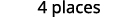Write as a product with a power of 10.$$\begin{array} {ll} {} &{3.7\times 10^4 } \\ {\text{Check:}} &{3.7 \times 10,000 } \\ {} &{37,000} \\ \end{array}$$The original number, 0.0052, is between 0 and 1 so we will have a negative power of 10. 0.0052 Move the decimal point to get 5.2, a number between 1 and 10.Count the number of decimal places the point was moved.Write as a product with a power of 10.$$\begin{array} {ll} {\text{Check:}} &{5.2\times10^{−3}} \\ {} &{5.2\times\dfrac{1}{10^3}} \\ {} &{5.2\times\dfrac{1}{1000}} \\ {} &{5.2\times 0.001} \\ {} &{0.0052} \\ \end{array}$$##### EXAMPLE $$\PageIndex{32}$$

Write in scientific notation: ⓐ 96,000 ⓑ 0.0078.

ⓐ $$9.6\times 10^4$$ ⓑ $$7.8\times 10^{−3}$$

##### EXAMPLE $$\PageIndex{33}$$

Write in scientific notation: ⓐ 48,300 ⓑ 0.0129.

ⓐ $$4.83\times10^4$$
ⓑ $$1.29\times10^{−2}$$

How can we convert from scientific notation to decimal form? Let’s look at two numbers written in scientific notation and see.

$\begin{array} {lll} {9.12\times10^4} &{} &{9.12\times10^{−4}} \\ {9.12\times10,000} &{} &{9.12\times0.0001} \\ {91,200} &{} &{0.000912} \\ \nonumber \end{array}$

If we look at the location of the decimal point, we can see an easy method to convert a number from scientific notation to decimal form.In both cases the decimal point moved 4 places. When the exponent was positive, the decimal moved to the right. When the exponent was negative, the decimal point moved to the left.

##### DEFINITION: CONVERT SCIENTIFIC NOTATION TO DECIMAL FORM.
1. Determine the exponent, $$n$$, on the factor 10.
2. Move the decimal $$n$$ places, adding zeros if needed.
• If the exponent is positive, move the decimal point $$n$$ places to the right.
• If the exponent is negative, move the decimal point $$|n|$$ places to the left.
3. Check.
##### EXAMPLE $$\PageIndex{34}$$

Convert to decimal form: ⓐ $$6.2\times10^3$$ ⓑ $$−8.9\times 10^{−2}$$.Determine the exponent, $$n$$, on the factor 10. The exponent is 3. Since the exponent is positive, move the decimal point 3 places to the right.Add zeros as needed for placeholders.Determine the exponent, $$n$$, on the factor 10. The exponent is −2.−2. Since the exponent is negative, move the decimal point 2 places to the left.Add zeros as needed for placeholders.##### EXAMPLE $$\PageIndex{35}$$

Convert to decimal form: ⓐ $$1.3\times 10^3$$ ⓑ $$−1.2\times 10^{−4}$$.

ⓐ 1,300 ⓑ $$−0.00012$$

##### EXAMPLE $$\PageIndex{36}$$

Convert to decimal form: ⓐ $$−9.5\times 10^4$$ ⓑ $$7.5\times 10^{−2}$$.

ⓐ $$−950,000$$ ⓑ 0.075

When scientists perform calculations with very large or very small numbers, they use scientific notation. Scientific notation provides a way for the calculations to be done without writing a lot of zeros. We will see how the Properties of Exponents are used to multiply and divide numbers in scientific notation.

##### EXAMPLE $$\PageIndex{37}$$

Multiply or divide as indicated. Write answers in decimal form: ⓐ $$(−4\times10^5)(2\times10^{−7})$$ ⓑ $$\dfrac{9\times10^3}{3\times10^{−2}}$$.

$$\begin{array} {ll} {} &{(−4\times10^5)(2\times10^{−7})} \\ {\text{Use the Commutative Property to rearrange the factors.}} &{−4·2·10^5·10^{−7}} \\ {\text{Multiply.}} &{−8\times10^{−2}} \\ {} &{} \\ {\text{Change to decimal form by moving the decimal two}} &{} \\ {\text{places left.}} &{−0.08} \\ \end{array}$$

$$\begin{array} {ll} {} &{\dfrac{9\times10^3}{9\times10^{−2}}} \\ {\text{Separate the factors, rewriting as the product of two}} &{} \\ {\text{fractions.}} &{\dfrac{9}{3}\times\dfrac{10^3}{10^{−2}}} \\ {\text{Divide.}} &{3\times10^5} \\ {\text{Change to decimal form by moving the decimal five}} &{} \\ {\text{places right.}} &{300,000} \\ \end{array}$$

##### EXAMPLE $$\PageIndex{38}$$

Multiply or divide as indicated. Write answers in decimal form:

ⓐ $$(−3\times10^5)(2\times10^{−8})$$ ⓑ $$\dfrac{8\times10^2}{4\times10^{−2}}$$.

ⓐ $$−0.006$$ ⓑ 20,000

##### EXAMPLE $$\PageIndex{39}$$

Multiply or divide as indicated. Write answers in decimal form:

ⓐ $$(−3\times10^{−2})(3\times10^{−1})$$ ⓑ $$\dfrac{8\times10^4}{2\times10^{−1}}$$.

ⓐ $$−0.009$$ ⓑ 400,000

Access these online resources for additional instruction and practice with using multiplication properties of exponents.

• Properties of Exponents
• Negative exponents
• Scientific Notation

## Key Concepts

• Exponential NotationThis is read $$a$$ to the $$m^{th}$$ power.
In the expression $$a^m$$, the exponent $$m$$ tells us how many times we use the base $$a$$ as a factor.
• Product Property for Exponents
If $$a$$ is a real number and $$m$$ and $$n$$ are integers, then

$a^m·a^n=a^{m+n} \nonumber$

To multiply with like bases, add the exponents.
• Quotient Property for Exponents
If $$a$$ is a real number, $$a\neq 0$$, and $$m$$ and $$n$$ are integers, then

$\begin{array} {lllll} {\dfrac{a^m}{a^n}=a^{m−n},} &{m>n} &{\text{and}} &{\dfrac{a^m}{a^n}=\dfrac{1}{a^{n−m}},} &{n>m}\\ \nonumber \end{array}$

• Zero Exponent
• If $$a$$ is a non-zero number, then $$a^0=1$$.
• If $$a$$ is a non-zero number, then $$a$$ to the power of zero equals $$1$$.
• Any non-zero number raised to the zero power is $$1$$.
• Negative Exponent
• If $$n$$ is an integer and $$a\neq 0$$, then $$a^{−n}=\dfrac{1}{a^n}$$ or $$\dfrac{1}{a^{−n}}=a^n$$.
• Quotient to a Negative Exponent Property
If $$a$$ and $$b$$ are real numbers, $$a\neq 0$$, $$b\neq 0$$ and $$n$$ is an integer, then

$(ab)^{−n}=(ba)^n\nonumber$

• Power Property for Exponents
If $$a$$ is a real number and $$m$$ and $$n$$ are integers, then

$(a^m)^n=a^{m·n}\nonumber$

To raise a power to a power, multiply the exponents.
• Product to a Power Property for Exponents
If $$a$$ and $$b$$ are real numbers and $$m$$ is a whole number, then

$(ab)^m=a^mb^m \nonumber$

To raise a product to a power, raise each factor to that power.
• Quotient to a Power Property for Exponents
If $$a$$ and $$b$$ are real numbers, $$b\neq0$$, and $$m$$ is an integer, then

$\left(\dfrac{a}{b}\right)^m=\dfrac{a^m}{b^m} \nonumber$

To raise a fraction to a power, raise the numerator and denominator to that power.
• Summary of Exponent Properties
If $$a$$ and $$b$$ are real numbers, and $$m$$ and $$n$$ are integers, then
Property Description
Product Property $$a^m·a^n=a^{m+n}$$
Power Property $$(a^m)^n=a^{m·n}$$
Product to a Power $$(ab)^n=a^nb^n$$
Quotient Property $$\dfrac{a^m}{a^n}=a^{m−n}, a\neq 0$$
Zero Exponent Property $$a^0=1,a\neq 0$$
Quotient to a Power Property: $$\left(\dfrac{a}{b}\right)^m=\dfrac{a^m}{b^m}, b\neq 0$$
Properties of Negative Exponents $$a^{−n}=\dfrac{1}{a^n}$$ and $$\dfrac{1}{a^{−n}}=a^n$$
Quotient to a Negative Exponent $$\left(\dfrac{a}{b}\right)^{−n}=\left(\dfrac{b}{a}\right)^n$$
• Scientific Notation
A number is expressed in scientific notation when it is of the form

$a\space\times\space10^n \text{ where }1\leq a<10\text{ and } n \text{ is an integer.} \nonumber$

• How to convert a decimal to scientific notation.
1. Move the decimal point so that the first factor is greater than or equal to 1 but less than 10.
2. Count the number of decimal places, $$n$$, that the decimal point was moved.
3. Write the number as a product with a power of 10. If the original number is.
• greater than 1, the power of 10 will be $$10^n$$.
• between 0 and 1, the power of 10 will be $$10^{−n}$$.
4. Check.
• How to convert scientific notation to decimal form.
1. Determine the exponent, $$n$$, on the factor 10.
2. Move the decimal $$n$$ places, adding zeros if needed.
• If the exponent is positive, move the decimal point $$n$$ places to the right.
• If the exponent is negative, move the decimal point $$|n|$$ places to the left.
3. Check.

## Glossary

Product Property
According to the Product Property, $$a$$ to the $$m$$ times $$a$$ to the $$a$$ equals $$a$$ to the $$m$$ plus $$n$$.
Power Property
According to the Power Property, $$a$$ to the $$m$$ to the $$n$$ equals $$a$$ to the $$m$$ times $$n$$.
Product to a Power
According to the Product to a Power Property, $$a$$ times $$b$$ in parentheses to the $$m$$ equals $$a$$ to the $$m$$ times $$b$$ to the $$m$$.
Quotient Property
According to the Quotient Property, $$a$$ to the $$m$$ divided by $$a$$ to the $$n$$ equals $$a$$ to the $$m$$ minus $$n$$ as long as $$a$$ is not zero.
Zero Exponent Property
According to the Zero Exponent Property, $$a$$ to the zero is $$1$$ as long as $$a$$ is not zero.
Quotient to a Power Property
According to the Quotient to a Power Property, $$a$$ divided by $$b$$ in parentheses to the power of $$m$$ is equal to $$a$$ to the $$m$$ divided by $$b$$ to the $$m$$ as long as $$b$$ is not zero.
Properties of Negative Exponents
According to the Properties of Negative Exponents, $$a$$ to the negative $$n$$ equals $$1$$ divided by $$a$$ to the $$n$$ and $$1$$ divided by $$a$$ to the negative $$n$$ equals $$a$$ to the $$n$$.
Quotient to a Negative Exponent
Raising a quotient to a negative exponent occurs when $$a$$ divided by $$b$$ in parentheses to the power of negative $$n$$ equals $$b$$ divided by $$a$$ in parentheses to the power of $$n$$.

This page titled 5.3: Properties of Exponents and Scientific Notation is shared under a CC BY 4.0 license and was authored, remixed, and/or curated by OpenStax via source content that was edited to the style and standards of the LibreTexts platform; a detailed edit history is available upon request.